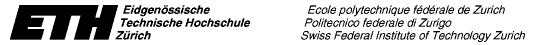# ETH Risk-Day

### Mini-Conference on Risk Management in Finance and Insurance

Location: ETH Main Building, Rämistr. 101, Lecture Room D 1.2

Time: Friday, September 25, 1998, 8.45 - 16.30

Conference program and abstracts are available as:

## Program

 8.45 - 9.00 Prof. Paul Embrechts (Dept. of Math., ETH Zürich) Financial and Insurance Mathematics at ETH Zürich Abstract: We give a short overview of the activities in Financial and Insurance Mathematics at ETH Zürich. 9.00 - 9.40 Prof. Freddy Delbaen (Dept. of Math., ETH Zürich) A Mathematical Problem Related to the Definition of Risk Measures Abstract: The definition of coherent risk measures as introduced by Artzner, Delbaen, Eber and Heath for finite probability spaces should be extended to the space of all random variables (e.g. normally distributed ones). However, there are mathematical obstacles that forbid "direct" generalisations. We will discuss a mathematical problem related to such an extension. The extra conditions we have to put on risk measures have straightforward interpretations in risk theory. 9.40 - 10.20 Dr. Gerold Studer (KPMG and IfOR, ETH Zürich) Maximum Loss for Market Risk Measurement Abstract: Effective risk management requires adequate risk measurement. A basic problem herein is the quantification of market risk: what is the overall effect on a portfolio if market rates change? The concept of Maximum Loss (ML) is introduced as a method for identifying the worst outcome in a given scenario space, called "trust region". The ML approach does not only determine a risk figure, but also the worst case scenario. The knowledge of this scenario builds a basis for a proactive risk management. In contrast to Value-at-Risk (VaR), ML can be calculated efficiently for nonlinear portfolios. An approach using successive quadratic approximations of the profit-and-loss function is presented and the characteristics of ML and VaR are compared to each other. 10.20 - 10.50 Coffee Break 10.50 - 11.30 Dr. Alexander McNeil (Dept. of Math., ETH Zürich) Estimation of Risk Measures in Financial Time Series: Combining Extreme Value Theory and Econometric Modelling Abstract: We propose a method for estimating VaR and related risk measures describing the tail of the conditional distribution of a heteroscedastic financial return series. Our approach combines quasi-maximum-likelihood fitting of GARCH models to estimate the current volatility and extreme value theory for estimating the tail of the innovation distribution of the GARCH model. We use our method to estimate conditional quantiles (VaR) and conditional expected shortfalls (the expected size of a return exceeding VaR). Using backtesting we show that our procedure gives better estimates than methods which ignore the heavy tails of the innovations or the stochastic nature of the volatility. With the help of our fitted models and a simulation approach we estimate the conditional quantiles of returns over multiple day horizons and find evidence of a power scaling law, where the power depends in a natural way on the current volatility level. 11.30 - 12.10 Dr. Rüdiger Frey (Dept. of Math., ETH Zürich) Risk Management for Derivatives under Stochastic Volatility Abstract: Stochastic volatility models have been introduced in order to deal with the well-known empirical deficiencies of the standard Black-Scholes model. These models are incomplete, i.e., a perfect replication of the payoff of derivatives by dynamic trading is not always possible. This raises important questions for the pricing and hedging of derivative securities. We discuss the superhedging of derivatives in a stochastic volatility model under the additional assumption that the volatility follows a bounded process. We characterize the value process of a superhedging strategy by an optimal-stopping problem which is similar to the optimal-stopping problem that arises in the pricing of American options. We relate our work to PDE-characterizations of superhedging strategies and illustrate our approach by examples and simulations. 12.10 - 14.00 Lunch Break 14.00 - 14.40 Dr. Uwe Schmock (Dept. of Math., ETH Zürich) Model Risk and Securitization Problems in Insurance Abstract: When fitting data and pricing financial instruments, the choice of the underlying model can significantly predetermine the corresponding results. This model risk is illustrated by the WinCAT coupons "Hail" of the Winterthur Insurance convertible bond, where many different models are consistent with the available historical data. The wide spectrum of different results arising from these models is presented. For selected models, the consistency and the dispersion are analyzed by simulation studies. 14.40 - 15.10 Daniel Straumann (RiskLab, ETH Zürich) Tempting Fallacies in the Use of Correlation Abstract: Linear correlation is extensively used in actuarial and financial mathematics. However, there are pitfalls and frequent misinterpretations which do not seem to be widely known. In this talk we discuss some problems related to the use of correlation. 15.10 - 15.30 Coffee Break 15.30 - 16.00 Michael Studer (Dept. of Math., ETH Zürich) Saddlepoint Approximations in Finance Abstract: Under the assumptions of the Black-Scholes model most quantities of financial interest can be computed analytically. But in many cases the assumptions of this model are too far from reality and one wants to use more realistic (and hence more complex) models such as continuous-time stochastic volatility models or models in discrete time which exhibit changes in variance such as ARCH/GARCH models. Most of these models have one major drawback in common: the marginal distribution of asset prices is not known analytically, which implies that time-consuming simulations have to be carried out for the computation of option prices or quantiles. There are several possible ways to "keep things analytic". We present two density approximations and discuss some applications in finance for the saddlepoint approximation. 16.00 - 16.30 Damir Filipovic (Dept. of Math., ETH Zürich) On the Consistency of Exponential-Polynomial Families for Forward Rate Curves Abstract: We discuss problems which arise from using a set of exponential-polynomial-type functions to model the instantaneous forward rate curve. A particular example among these is the so called Nelson-Siegel family.

### General Information

Participation is free, and there is no official registration. Everyone is welcome, practitioners are especially encouraged to attend. We have not made any special arrangements for lunch since there are sufficient possibilities on site, in particular the Dozentenfoyer.

Local Organizers: Uwe Schmock, Rüdiger Frey, Paul Embrechts

Conference Secretary: Mrs G. Baltes, HG G37.2, Phone 01/632 34 00, E-mail: baltes@math.ethz.ch

Following Risk Days: 1999, 2000, 2001, 2002, 2003, 2004